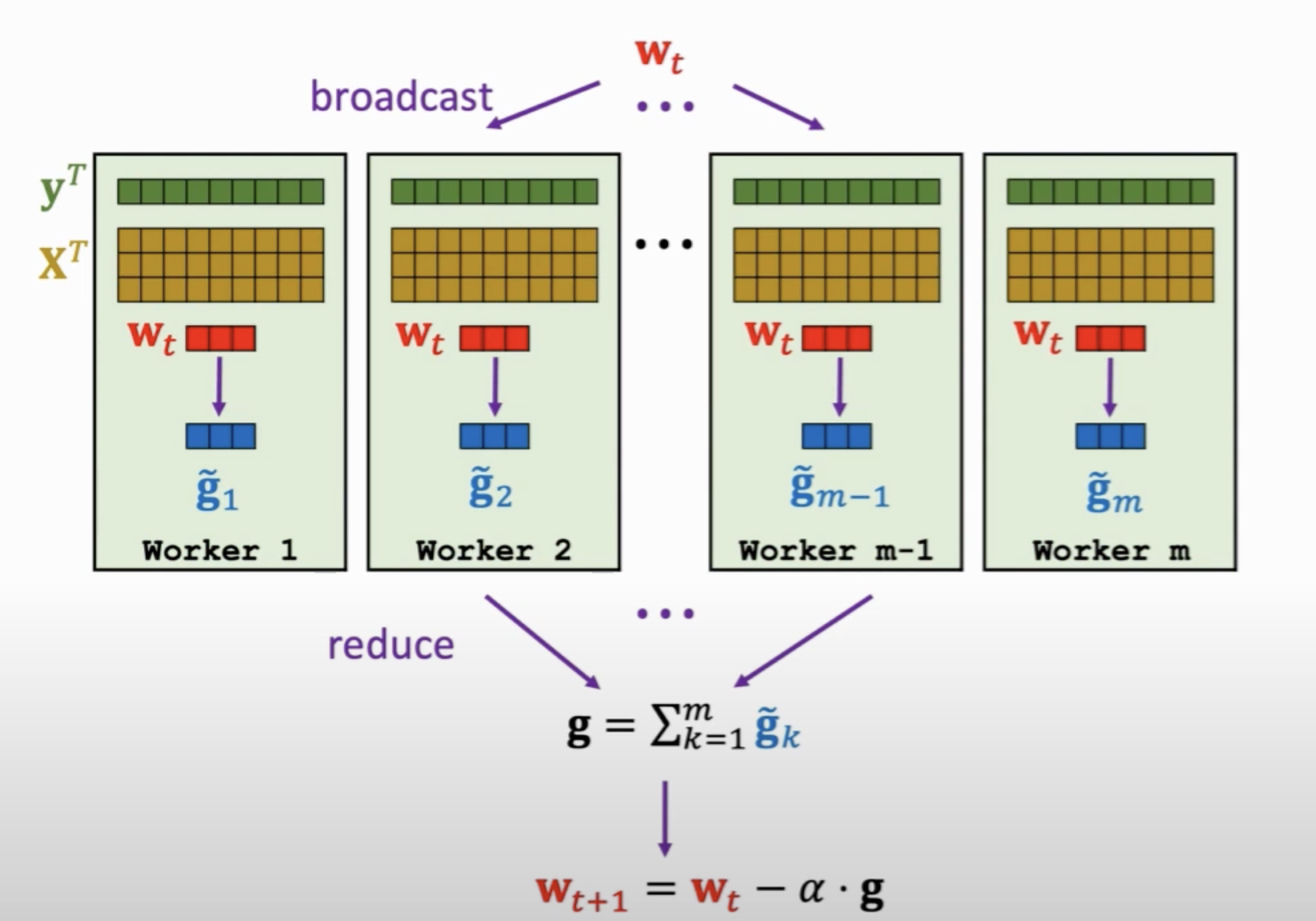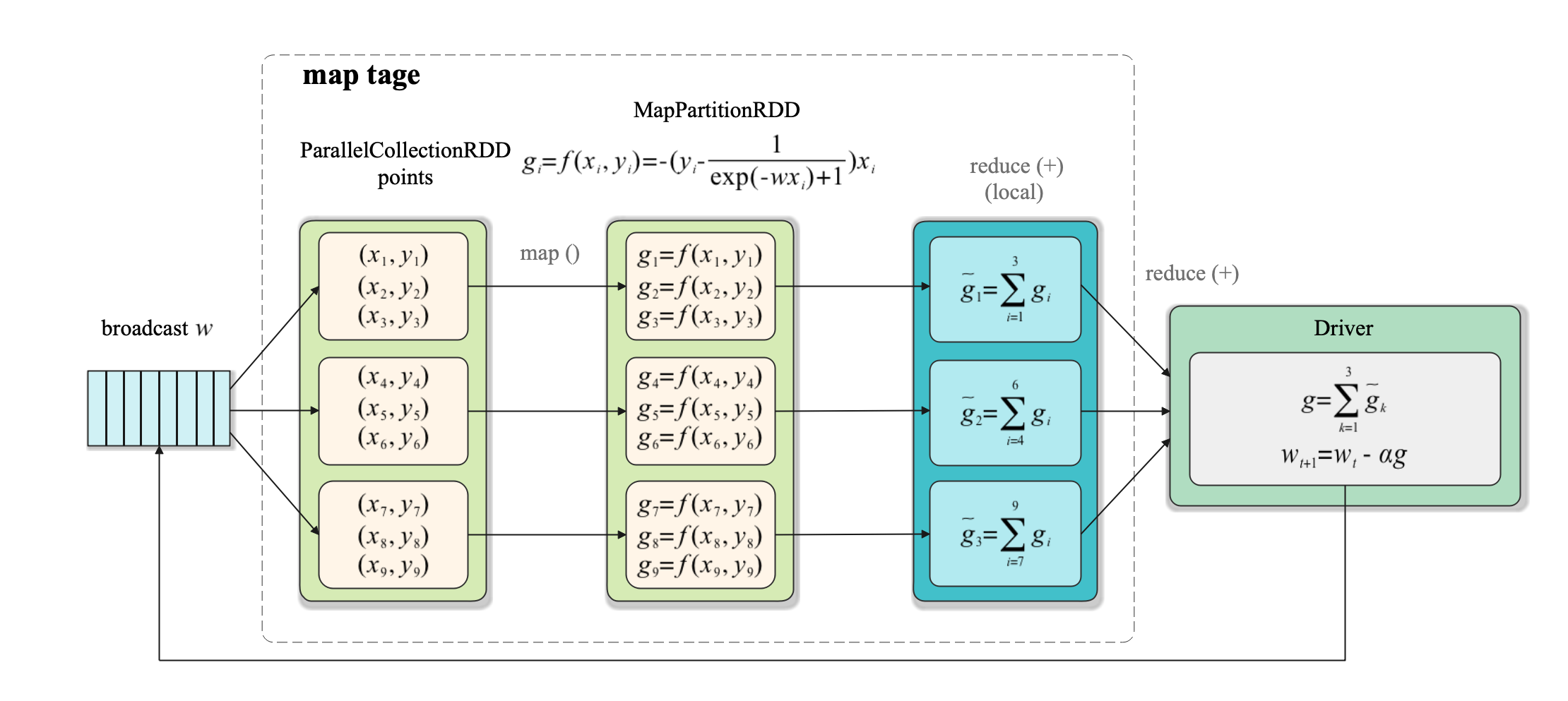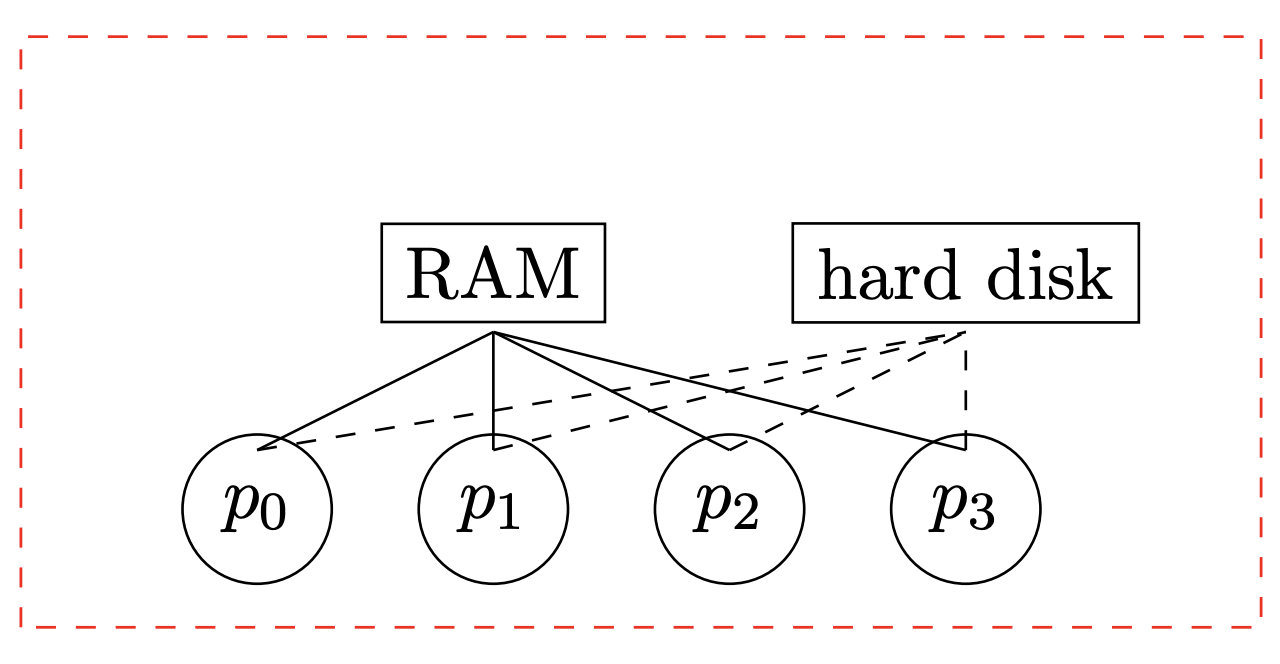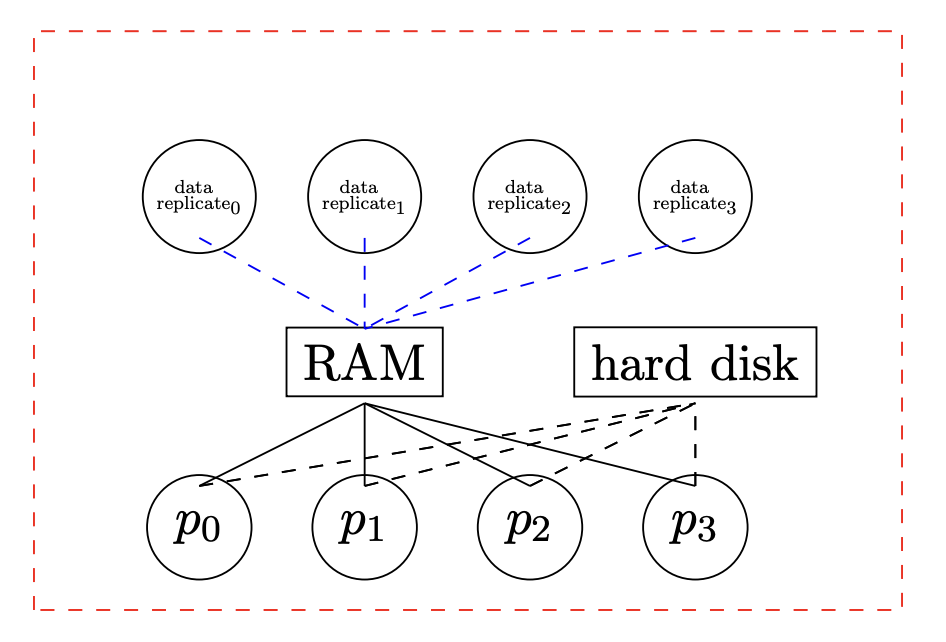# 分布式机器学习：逻辑回归的并行化实现（PySpark）

## 1. 梯度计算式导出

$\mathcal{l}(w) = \sum_{i=1}^{N}\left[y_{i} \log \pi_{w}\left(x_{i}\right)+\left(1-y_{i}\right) \log \left(1-\pi_w\left(x_{i}\right)\right)\right]$

\begin{aligned} \pi_w(x) = p(y=1 \mid x; w) =\frac{1}{1+\exp \left(-w^{T} x\right)} \end{aligned}

$\mathcal{l}(w) = -\sum_{i=1}^N(y_i w^T x_i - \log(1 + \exp(w^T x_i)))$

$\nabla_w{\mathcal{l}(w)} = -\sum_{i=1}^N(y_i - \frac{1}{\exp(-w^Tx)+1})x_i$

## 2. 基于Spark的并行化实现• map阶段： 各task运行map()函数对每个样本$$(x_i, y_i)$$计算梯度$$g_i$$， 然后对每个样本对应的梯度运行进行本地聚合，以减少后面的数据传输量。如第1个task执行reduce()操作得到$$\widetilde{g}_1 = \sum_{i=1}^3 g_i$$ 如下图所示：

• reduce阶段：使用reduce()将所有task的计算结果收集到Driver端进行聚合，然后进行参数更新。## 3. PySpark实现代码

PySpark的完整实现代码如下：

from sklearn.datasets import load_breast_cancer
import numpy as np
from pyspark.sql import SparkSession
from sklearn.model_selection import train_test_split
from sklearn.metrics import accuracy_score

n_slices = 3  # Number of Slices
n_iterations = 300  # Number of iterations
alpha = 0.01  # iteration step_size

def logistic_f(x, w):
return 1 / (np.exp(-x.dot(w)) + 1)

def gradient(point: np.ndarray, w: np.ndarray) -> np.ndarray:
""" Compute linear regression gradient for a matrix of data points
"""
y = point[-1]    # point label
x = point[:-1]   # point coordinate
# For each point (x, y), compute gradient function, then sum these up
return - (y - logistic_f(x, w)) * x

if __name__ == "__main__":

D = X.shape
X_train, X_test, y_train, y_test = train_test_split(
X, y, test_size=0.3, random_state=0)
n_train, n_test = X_train.shape, X_test.shape

spark = SparkSession\
.builder\
.appName("Logistic Regression")\
.getOrCreate()

matrix = np.concatenate(
[X_train, np.ones((n_train, 1)), y_train.reshape(-1, 1)], axis=1)

points = spark.sparkContext.parallelize(matrix, n_slices).cache()

# Initialize w to a random value
w = 2 * np.random.ranf(size=D + 1) - 1
print("Initial w: " + str(w))

for t in range(n_iterations):
print("On iteration %d" % (t + 1))
w -= alpha * g

y_pred = logistic_f(np.concatenate(
[X_test, np.ones((n_test, 1))], axis=1), w)
pred_label = np.where(y_pred < 0.5, 0, 1)
acc = accuracy_score(y_test, pred_label)
print("iterations: %d, accuracy: %f" % (t, acc))

print("Final w: %s " % w)
print("Final acc: %f" % acc)

spark.stop()


Initial w: [-0.0575882   0.79680833  0.96928013  0.98983501 -0.59487909 -0.23279241
-0.34157571  0.93084048 -0.10126002  0.19124314  0.7163746  -0.49597826
-0.50197367  0.81784642  0.96319482  0.06248513 -0.46138666  0.76500396
0.30422518 -0.21588114 -0.90260279 -0.07102884 -0.98577817 -0.09454256
0.07157487  0.9879555   0.36608845 -0.9740067   0.69620032 -0.97704433
-0.30932467]


Final w: [ 8.22414803e+02  1.48384087e+03  4.97062125e+03  4.47845441e+03
7.71390166e+00  1.21510016e+00 -7.67338147e+00 -2.54147183e+00
1.55496346e+01  6.52930570e+00  2.02480712e+00  1.09860082e+02
-8.82480263e+00 -2.32991671e+03  1.61742379e+00  8.57741145e-01
1.30270454e-01  1.16399854e+00  2.09101988e+00  5.30845885e-02
8.28547658e+02  1.90597805e+03  4.93391021e+03 -4.69112527e+03
1.10030574e+01  1.49957834e+00 -1.02290791e+01 -3.11020744e+00
2.37012097e+01  5.97116694e+00  1.03680530e+02]
Final acc: 0.923977


## 4.关于冗余存储的反思

map(lambda point: gradient(point, w)).reduce(add)# Initialize w to a random value
w = 2 * np.random.ranf(size=D + 1) - 1
print("Initial w: " + str(w))

for t in range(n_iterations):
print("On iteration %d" % (t + 1))

w -= alpha * g


## 5.关于聚合效率的反思

treeAggregate()函数原型如下：

RDD.treeAggregate(zeroValue, seqOp, combOp, depth=2)


depth为聚合树的深度。

g = points.map(lambda point: gradient(point, w_br.value))\


## 6. 复杂度和通信时间分析

### 6.2 通信时间

$2\text{log}_2(p)(L + \frac{m}{B})$